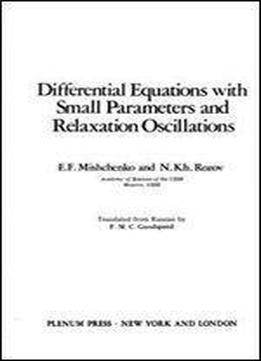# Differential Equations With Small Parameters And Relaxation Oscillations (mathematical Concepts And Methods In Science And Engineering) by E. Mishchenko / 1980 / English / PDF

The monograph presents a method of constructing asymptotic solutions of normal autonomous systems of ordinary differential equations with a small parameter multiplying some of the derivatives. This method allows one to obtain asymptotic representations for trajectories of such systems on any time interval, to compute periodic solutions and to find various quantities that characterise a solution (in particular, the period of a periodic solution). The questions considered in the book are of interest in the study of some mechanical, physical and technological problems as, for example, in the theory of relaxation oscillations.

views: 242